Previous Article                                                                         Next Article

In this article we will continue our discussion of the Platonic solids by moving into the topic of duals and the sacred number canon.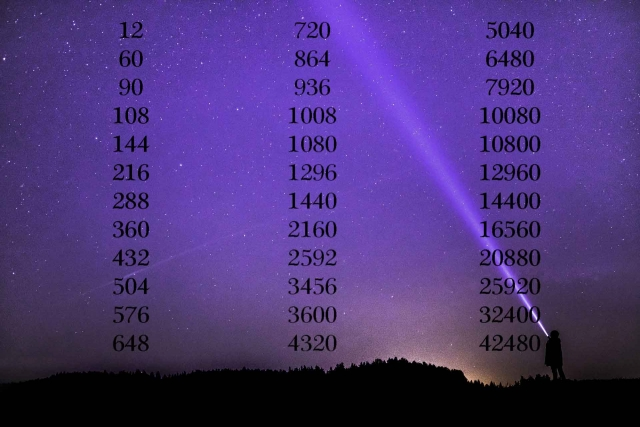We first discussed the sacred number canon way back in Article 4.  The Sacred Number canon deals with numbers that are significant geometrically and astronomically.  This means that the numbers are associated with angles of the Platonic solids and astronomical cycles such as the Precession of the Equinoxes.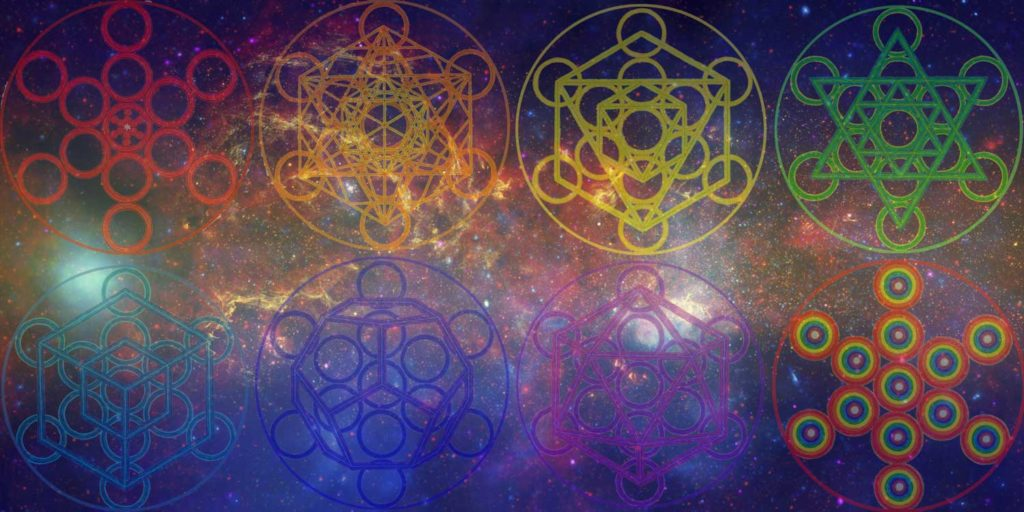Platonic Solid Duals

Duals are two polyhedra whose faces and vertices correspond perfectly.

This illustrates the Principle of Inversion.

As a Platonic solid oscillates it can turn inside out.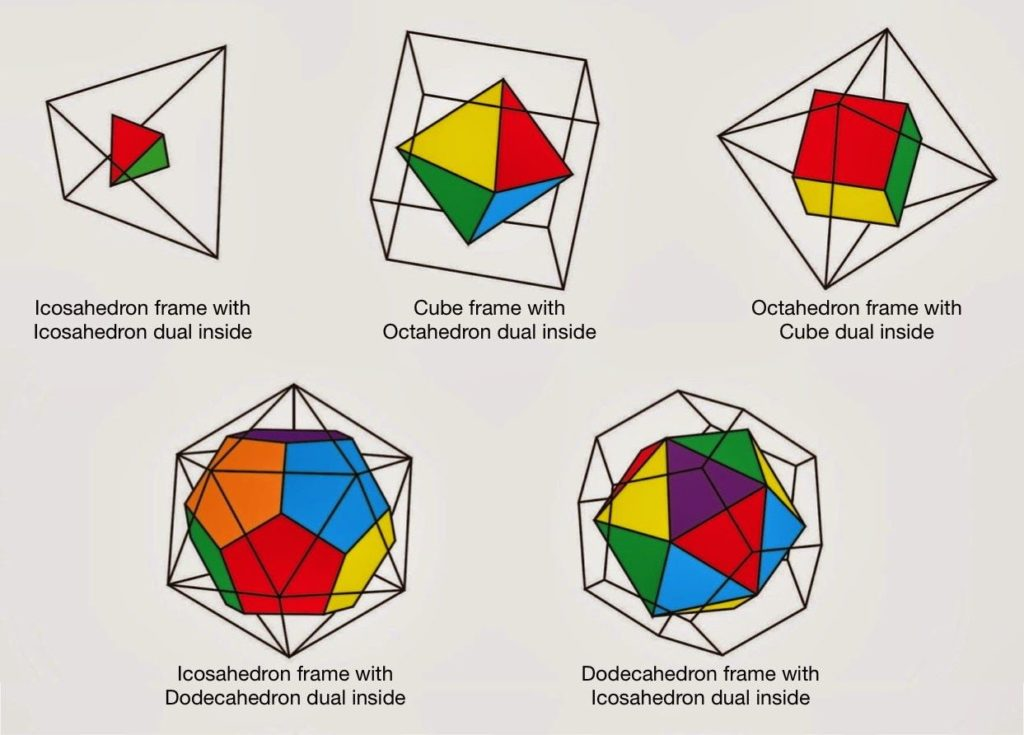To find the dual:

• Define the midpoint of each edge. Connect the points in 3D space.
• Or join the face centers:  To do so, define the midpoint of each face.  Connect the center of each face with a line.

The following are duals:

• Octahedron and Cube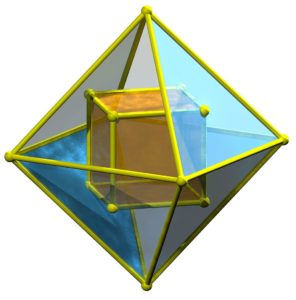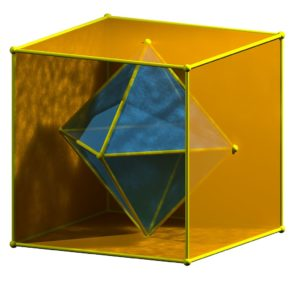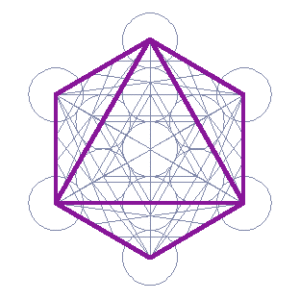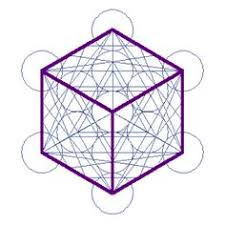• Icosahedron and Dodecahedron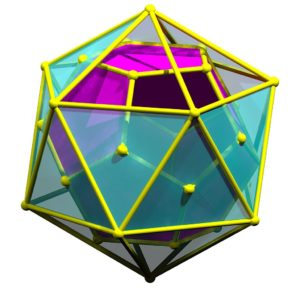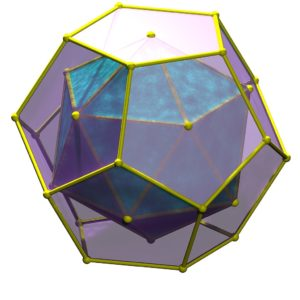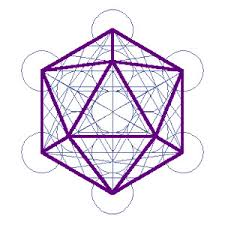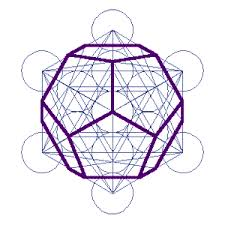“Not only does the projection of the internal radii of the icosahedron form the edges of the dodecahedron but reciprocally the projected radius of the dodecahedron produces the edges of the icosahedron.  This alternating projection of one form out of the other is only graphically indicated here, but is geometrically a fact.”1• Tetrahedron is its own dual (self-dual).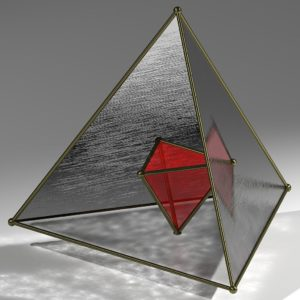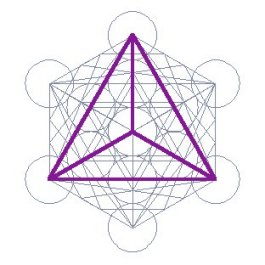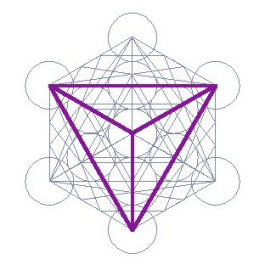• Only the tetrahedron is its own dual. When it merges with its dual it forms a star tetrahedron (a.k.a. the stellated octahedron, pictured below)Duals can be married with their edges touching at their midpoints to create compound polyhedra:

• Star Tetrahedron – This creates a stellated octahedron from two tetrahedra.  This is the balance of two tetrahedra.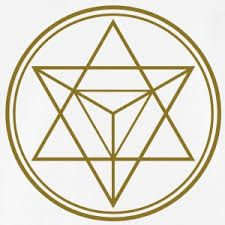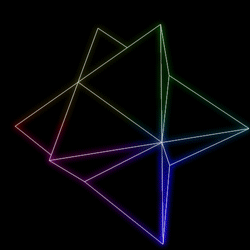• Cuboctahedron (vector equilibrium) – Balance of the cube and octahedron.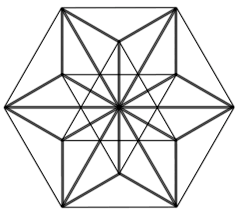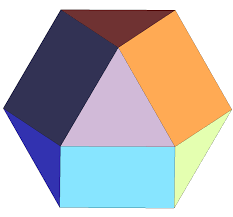• Icosidodecahedron – Balance of the icosahedron and dodecahedron.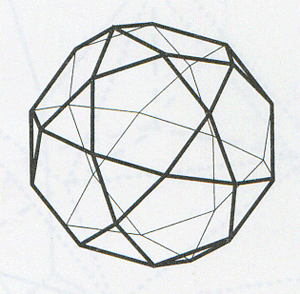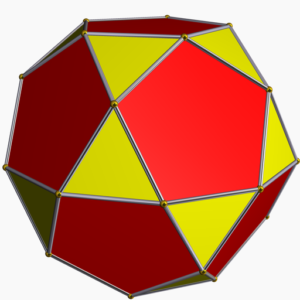The Platonic Solids and the Four Elements

As we saw in earlier articles, the sum of the angles of the four Platonic solids that represent Fire, Air, Earth & Water (the 4 Earthly Elements) equals the diameter of the Earth in miles (99.97% accuracy).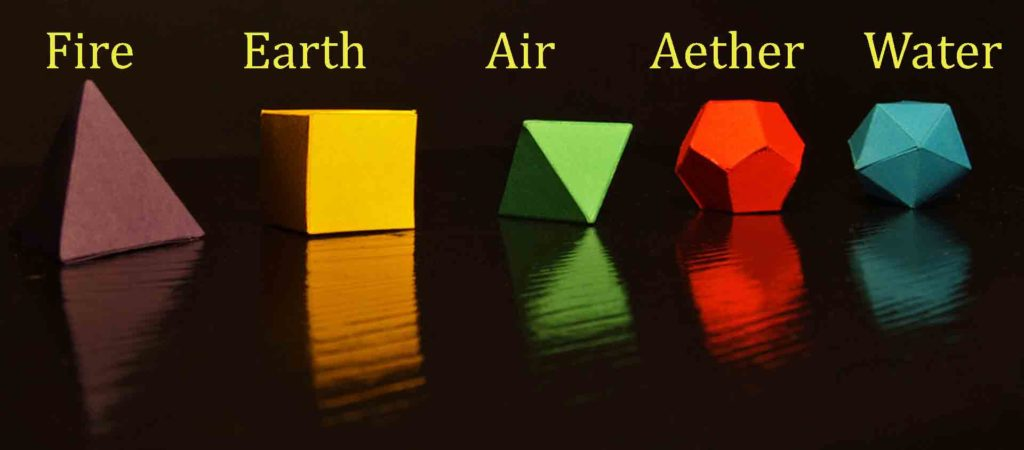Earth’s polar diameter in 2013 (NASA) = 7899.86 miles

The equatorial diameter = 7926.33 miles

The diameter from tropic of Cancer to tropic of Capricorn = 7920 miles

Sum of the angles of the tetrahedron, cube, octahedron, icosahedron (the 4 elements) = 7920º.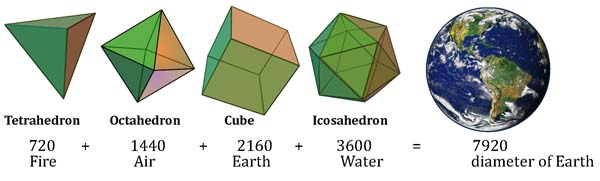Note that these numbers are always in flux due to seasonal, lunar, meteorological, and geological changes.

The Sum of the Angles of the Cube (2160°) = the diameter of the Moon in miles (99.99% accuracy).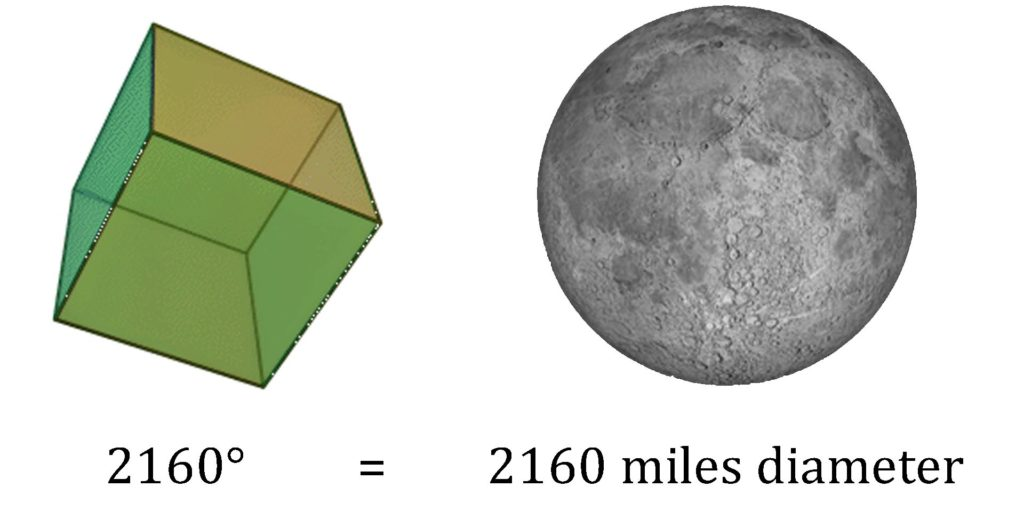2160 = the diameter of the Moon AND the length of 1 “Great Month” in the 25,920 year cycle of the Precession of the Equinoxes.

Time AND Space are determined by Geometry – and not just any Geometry, Platonic Solid geometry.

The sum of the angles of the tetrahedron (fire), octahedron (air) and dodecahedron (Aether/spirit) = 8640°.

8640 x 100 = 864,000 miles = the diameter of the Sun (99.95%)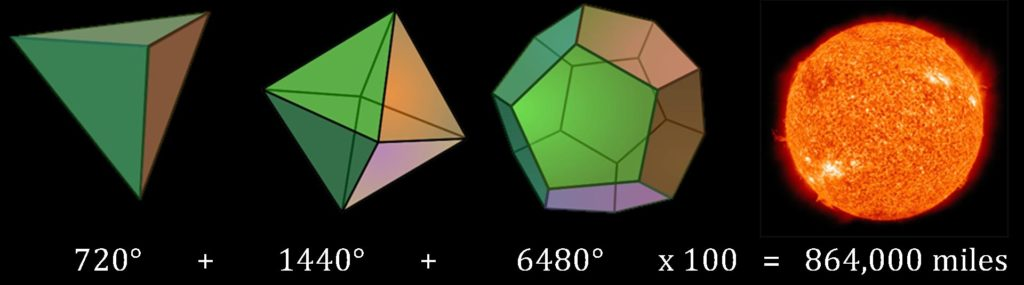Precessional Numbers & Platonic Solids

The following are the most important Precessional numbers.  These numbers are related to the cycles of the Precession of the Equinoxes (See Article 256).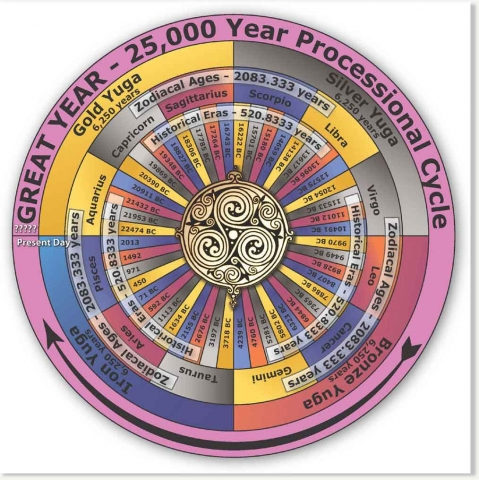12, 30, 36, 54, 72, 108, 144, 360, 540, 2160, 4320, 25920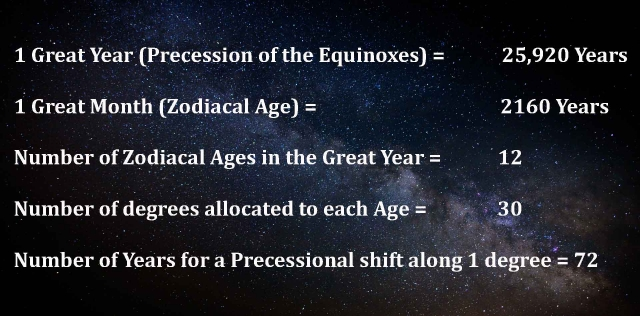12

• number of constellations in the Zodiac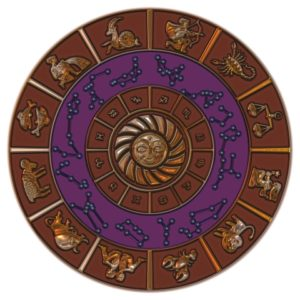• number of ages in one precessional cycle
• number of edges of the cube and octahedron
• number of vertices of the icosahedron & cuboctahedron
• number of faces of the dodecahedron & rhombic dodecahedron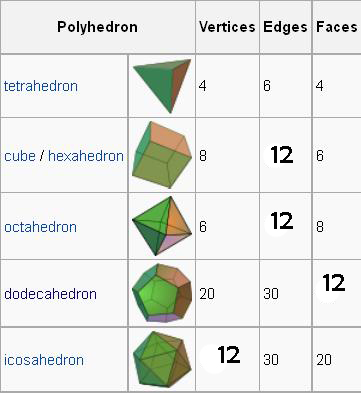30

• Number of degrees allocated along the ecliptic to each zodiacal constellation
• 360/12 constellations = 30° per age
• Number of edges of the Icosahedron & Dodecahedron36

• Number of years required for the equinoctial sun to complete a precessional shift of half a degree along the ecliptic
• The number of degrees in the interior angle of a regular pentagram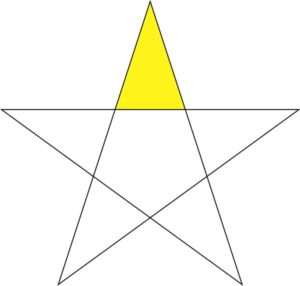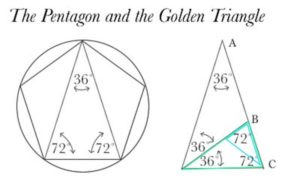• The number of edges in a truncated cube and truncated octahedron (Archimedean solids)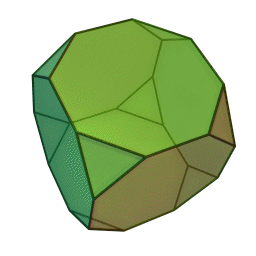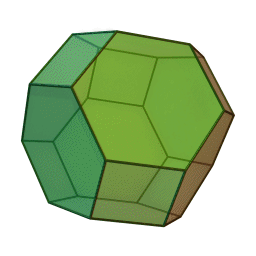• The sum of the integers from 1 to 36 = 666
• 1 + 2 + 3 + 4 + 5 + 6 + 7 + 8 + 9 + 10 + 11 + 12 + 13 + 14 + 15 + 16 + 17 + 18 + 19 + 20 + 21 + 22 + 23 + 24 + 25 + 26 + 27 + 28 + 29 + 30 + 31 + 32 + 33 + 34 + 35 + 36 = 666

54

• 72 x 36 = 108
• (108/2 = 54) which may then be multiplied by 10 and expressed as 540 (or as 54,000 or as 540,000 or as 5,400,000 and so on).
• The sine of a 54 degree angle is half the golden ratio
• 540 = the sum of angles in a regular pentagon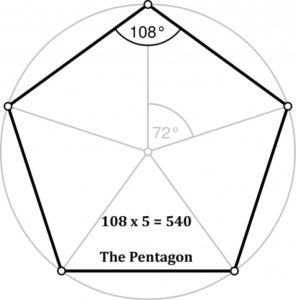72

• The number of years required for the equinoctial sun to complete a precessional shift of one degree along the ecliptic.  This means 72 years = 1 ‘day’ of Precession.
• 25920/360 = 72

• 72 = the exterior angles of a regular pentagon
• 72 = the number of edges in a truncated cuboctahedron & disdyakis dodecahedron

• 720 = sum of the angles of a tetrahedron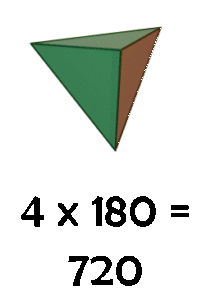• 720 = sum of angles of a hexagon (120 x 6 = 720)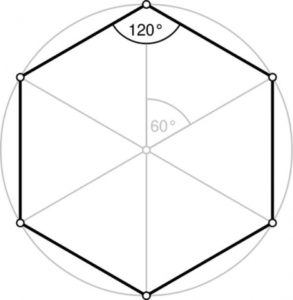• 720 x 2 = 1440 = sum of angles in a regular decagon, octahedron, and star tetrahedron
• 720 x 5 = 3600 = sum of angles in an icosahedron, cuboctahedron, truncated tetrahedron
• 720 x 6 = 4320 = sum of angles in a tetrakis hexahedron and triakis octahedron
• 720 x 9 = 6480 = sum of the angles of a dodecahedron; sum of angles of triangle thru decagon

• 720 x 11 = 7920 = Diameter of the Earth in miles and the sum of degrees in the rhombicuboctahedron, snub cube, truncated octahedron, and truncated cube

• 720 x 12 = 8640 = sum of angles in the trapezoidal icositetrahedron and disdyakis dodecahedron
• 8640 x 10 = 86400 = number of seconds in a day
• 8640 x 100 = 864000 = diameter of the Sun in miles

• 720 x 14 = 10080 = sum of angles in an icosidodecahedron
• 720 x 15 = 10800 = sum of angles in a pentakis dodecahedron, triakis icosahedron, and rhombic triacontahedron

• 720 x 18 = 12960 = sum of angles in a pentagonal icositetrahedron
• 720 x 20 = 14400 = sum of all five Platonic solids
• 720 x 23 = 16560 = sum of angles in a great rhombicuboctahedron
• 720 x 29 = 20880 = sum of the angles of the rhombicosidodecahedron, snub dodecahedron, truncated icosahedron, and truncated dodecahedron (All Archimedean solids)

• 720 x 45 = 32400 = sum of angles in a pentagonal hexecontahedron.
• 720 x 59 = 42480 = sum of angles in a truncated icosidodecahedron
• 720 x 210 = 151200 = sum of all Catalan solids
• 720 x 230 = 165600 = sum of all Archimedean solids

* The sum of the angles of all Platonic solids, Archimedean solids and Catalan solids are factors of 72!

Platonic solids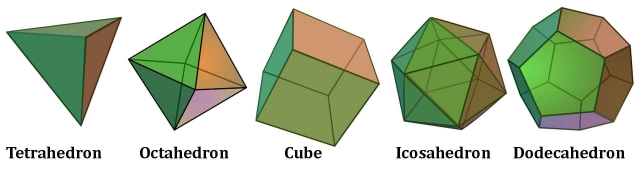Archimedean solids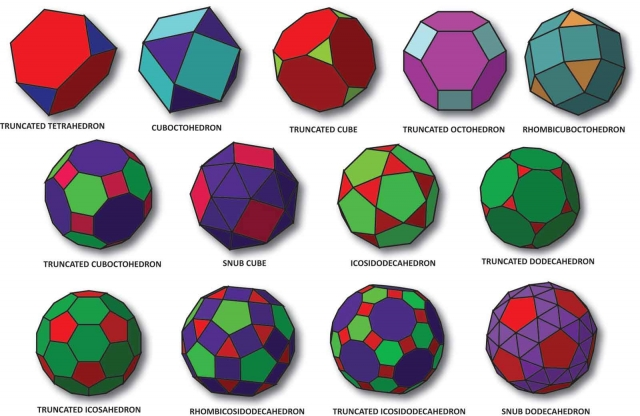Catalan solids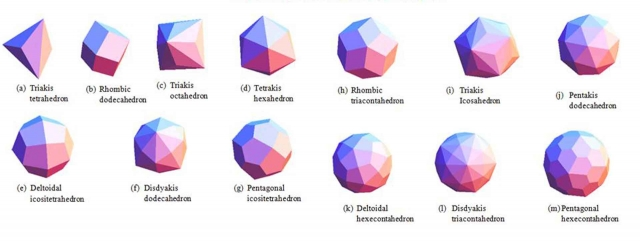108

• The pre-eminent number in the code is 72.  To this is frequently added 36, making 108, and it is permissible to multiply 108 by 100 to get 10,800.
• 108 = degrees in interior angles of a regular pentagon
• 1080 = sum of angles of an octagon (135 x 8 = 1080)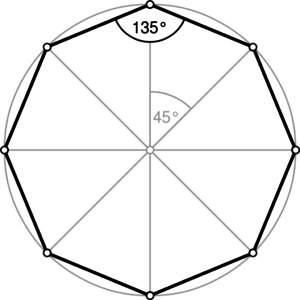144 = 12 x 12

• 1440 = sum of angles of a star tetrahedron (2 x 720 = 1440º)• 1440 = sum of angles of a octahedron• 1440 = sum of angles of a decagon (10 sides: 144 x 10 = 1440)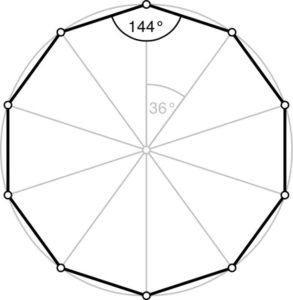360

• the total number of degrees in the ecliptic
• 360 degrees in a circle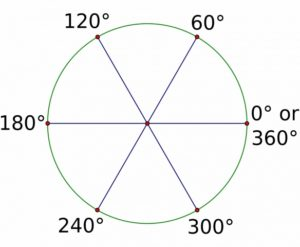• 360 = sum of angles of a square (90 x 4)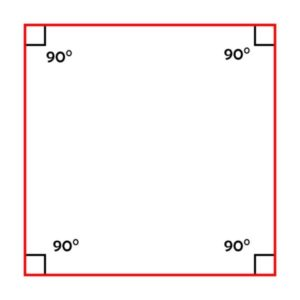• 3600 = sum of angles of an icosahedron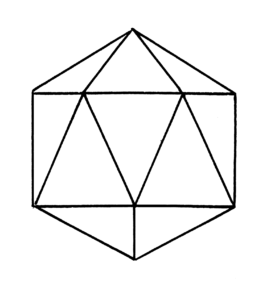2160

• 72 x 30 = 2160 = the number of years required for the sun to complete a passage of 30 degrees along the ecliptic (through one of the 12 zodiacal constellations).
• This means 1 zodiacal age = 2160 years.  That is, we spend 2160 years in Aries; 2160 in Pisces; 2160 in Aquarius…etc
• 2160 is sometimes multiplied by factors of 10 (to give 216,000, 2,160,000, and so on).
• 2160 = sum of interior angles of a cube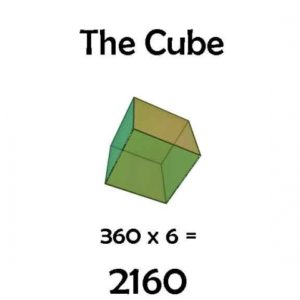4320

(2160 x 2) number of years required for the equinoctial sun to complete a precessional shift of 60 degrees (2 constellations).  Multiplying 4320 by factors of 10 gives 43,200 or 432,000 or 4,320,000, ad infinitum.

2160 (related to 432)

• 2160º =  sum of interior angles of cube
• 432/2 =  216
• 2160/2 = 1080º = octagon
• 2160/3 = 720º   = hexagon
• 2160/4 = 540º   = pentagon
• 2160/5 = 432
• 2160/6 = 360º   = square and circle

• 432,000 miles = radius of the Sun
• 4322 = 186,624 miles/second (speed of light)432 the only whole number when squared comes into .01% accuracy measuring the speed of light.

The official speed of light is 186,282 miles/second (a .01% difference).

25,920

• 25920 = 2160 x 12 (or 360 x 72)
• the number of years in one complete precessional cycle or ‘Great Year”, and thus the total number of years required to bring about the “Great Return”
• 25,920 = the average number of human breaths taken in one day.
• 25920 = The total number of degrees in the angles of a 64 tetrahedron grid (the Isotropic Vector Matrix)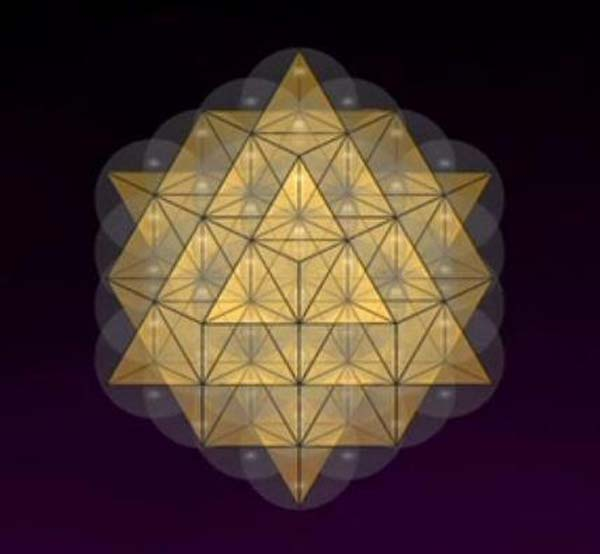1. Lawlor, Robert, Sacred Geometry: Philosophy & Practice, Thames & Hudson, 1982